# KSEEB Solutions for Class 6 Maths Chapter 14 Practical Geometry Ex 14.6

Students can Download Chapter 14 Practical Geometry Ex 14.6 Questions and Answers, Notes Pdf, KSEEB Solutions for Class 6 Maths helps you to revise the complete Karnataka State Board Syllabus and score more marks in your examinations.

## Karnataka State Syllabus Class 6 Maths Chapter 14 Practical Geometry Ex 14.6

Question 1.
Draw ∠POQ of measure 75° and find its line of symmetry.
Solution: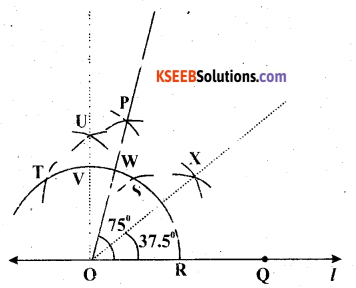The below given steps will be followed to construct an angle of 75° and its line of symmetry
(1) Draw a line l and mark two points O and Q on it, as shown in the figure. Draw an arc of convenient radius, while taking point O as centre. Let it intersect line l at R
(2) Taking R as centre and with the same radius as before, draw an arc intersecting the previously drawn arc at S.
(3) Taking S as centre and with the same radius as before, draw an arc intersecting the arc at T (see figure).
(4) Taking S and T as centre, draw an arc of same radius to intersect each other at U.
(5) Join OU. Let it intersect the arc at V. Now, taking S and V as centres, draw arcs with radius more than $$\frac{1}{2}$$SV. Let those intersect each other at P. Join OP, which is the ray making 75° with the line l.
(6) Let this ray be intersecting our major arc at point W. Now, taking R and W as centres draw arcs with radius more than $$\frac{1}{2}$$RW in the interior of angle of 75°. Let these be intersecting each other at X. Join OX.
OX is the line of symmetry for ∠POQ = 75°.Question 2.
Draw an angle of measure 147° and construct its bisector.
Solution:
The below given steps will be followed to construct an angle of 147° measure and its bisector.
(1) Draw a line l and mark a point O on it. Place the centre of the protractor at point O and the zero edge along line l.
(2) Mark a point A at 147°. Join O A. OA is the required ray making 147° with line l.
(3) Draw an arc of convenient radius, while taking point O as centre. Let it intersect both rays of angle 147° at point A and B.
(4) Taking A and B as centres, draw arcs of radius more than $$\frac{1}{2}$$AB in the interior of angle of 147°. Let those intersect each other at C. Join OC.
OC is the required bisector of 147° angle.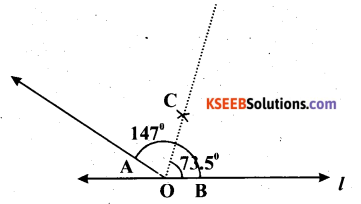a

Question 3.
Draw a right angle and construct its bisector.
Solution: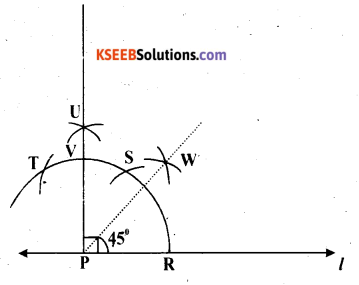The below given steps will be followed to construct a right angle and its bisector.
(1) Draw a line l and mark a point P on it. Draw an arc of convenient radius, while taking point P as centre. Let it intersect line l at R.
(2) Taking R as centre and with the same radius as before, draw an arc intersecting the previously drawn arc at S.
(3) Taking S as centre and with the same radius as before, draw an arc intersecting the arc at T (see figure).
(4) Taking S and T as centres, draw arcs of same radius to intersect each other at U.
(5) Join PU. PU is the required ray making 90° with line l. Let it intersect the major arc at point V.
(6) Now, taking R and V as centres, draw arcs with radius more than $$\frac{1}{2}$$RV to intersect each other at W. Join PW.
PW is the required bisector of this right angle.Question 4.
Draw an angle of measure 153° and divide it into four equal parts.
Solution: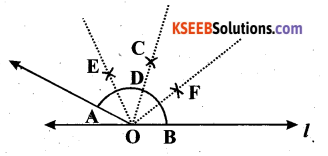The below given steps will be followed to construct an angle of 153° measure and its bisector.
(1) Draw a line l and mark a point O on it. Place the centre of the protractor at point O and the zero edge along line 1.
(2) Mark a point A at 153°. Join OA. O A is the required ray making 153° with line l.
(3) Draw an arc of convenient radius, while taking point O as centre. Let it intersect both rays of angle 153° at point A and B.
(4) Taking A and B as centres, draw arcs of radius more than $$\frac{1}{2}$$AB in the interior of angle of 153°. Let those intersect each other at G. Join OC.
(5) Let OC intersect the major arc at point D. Now, with radius more than $$\frac{1}{2}$$AD, draw arcs while taking A and D as centres, and D and B as centres. Let these be intersecting each other at point E and F respectively. Join OE, OF.
OF, OC, OE are the rays dividing 153° angle in 4 equal parts.

Question 5.
Construct with ruler and compasses, angles of following measures :
(a) 60°
(b) 30°
(c) 90°
(d) 120°
(e) 45°
(f) 135°
Solution:
(a) 60°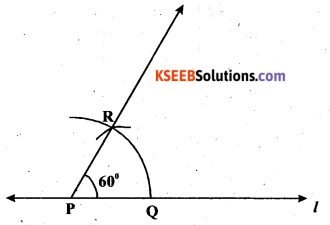The below given steps will be followed to construct an angle of 60°.
(1) Draw a line l and mark a point P on it. Now, taking P as centre and with a convenient radius, draw an arc of a circle which intersects line l at Q.
(2) Taking Q as centre and with the same radius as before, draw an arc intersecting the previously drawn arc at point R.
(3) Join PR Which is the required ray making 60° with line l.

(b) 30°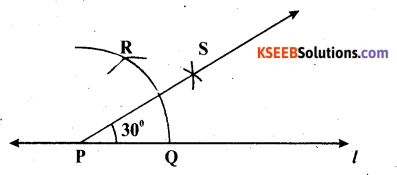The below given steps will be followed to construct an angle of 30°.
(1) Draw a line l and mark a point P on it. Now taking P as centre and with convenient radius, draw an arc of a circle which intersects line l at Q.
(2) Taking Q as centre and with the same radius as before, draw an arc intersecting the previously drawn arc at point R.
(3) Now, taking Q and R as centre and with radius more than RQ, draw arcs to intersect each other at S. Join PS which is the required ray making 30° with line l.(c) 90°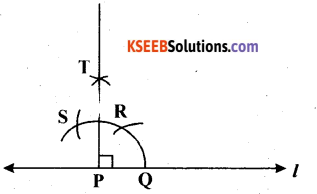The below given steps will be followed to construct an angle of 90°.
(1) Draw a line l and mark a point P on it. Now taking P as centre and with a convenient radius, draw an arc of a circle which intersects line l at Q.
(2) Taking Q as centre and with the same radius as before, draw an arc intersecting the previously drawn arc at R.
(3) Taking R as centre and with the same radius as before, draw an arc intersecting the arc at S (see figure).
(4) Taking R and S as centre, draw an arc of same radius to intersect each other at T.
(5) Join PT, which is the required ray making 900 with line l.

(d) 120°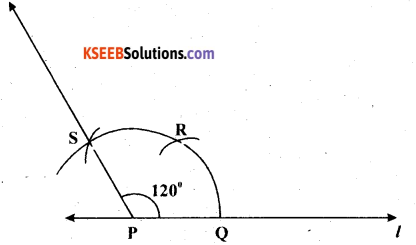The below given steps will be followed to construct an angle of 120°.
(1) Draw a line 1 and mark a point P on it. Now taking P as centre and with a convenient radius, draw an arc of a circle which intersects line l at Q.
(2) Taking Q as centre and with the same radius as before, draw an arc intersecting the previously drawn arc at R.
(3) Taking R as centre and with the same radius as before, draw an arc intersecting the arc at S (see figure).
(4) Join PS, which is the required ray making 120° with line l.

(e) 45°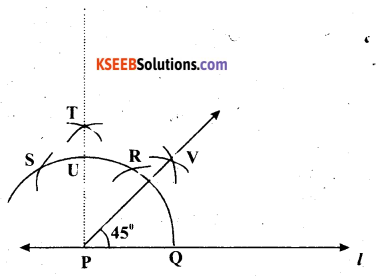The below given steps will be followed to construct an angle of 45°.
(1) Draw a line l and mark a point P on it. Now taking P as centre and with a convenient draw an arc of a circle which intersects line l at Q.
(2) Taking Q as centre and with the same radius as before, draw an arc intersecting the previously drawn arc at R.
(3) Taking R as centre and with the same radius as before, draw an arc intersecting the arc at S (see figure).
(4) Taking R and S as centres, draw arcs of same radius to intersect each other at T.
(5) Join PT. Let it intersect the major arc at point U.
(6) Taking Q andU as centres, draw arcs with radius more than QU to intersect each other at V. Join PV.
PV is the required ray making 45° with the given line l.

(f) 135°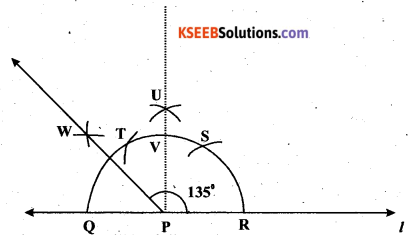The below given steps will be followed to construct an angle of 135°.
(1) Draw a line l and mark a point P on it. Now taking P as centre and with a convenient radius, draw a semi circle which intersects line l at Q and R.
(2) Taking R as centre and with the same radius as before, draw an arc intersecting the previously .drawn arc at S.
(3) Taking S as centre and with the same radius as before, draw an arc intersecting the arc at T (see figure).
(4) Taking S and T as centre, draw arcs of same radius to intersect each other at U.
(5) Join PU. Let it intersect the arc at V. Now taking Q and V as centres and with radius more than QV, draw arcs to intersect each other at W.
(6) Join PW which is the required ray making 135° with line l.Question 6.
Draw an angle of measure 45° and bisect it.
Solution: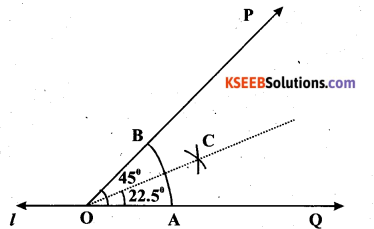The below given steps will be followed to construct.an angle of 45° and its bisector.
(1) ∠POQ of 45° measure can be formed on a line l by using the protractor.
(2) Draw an arc of a convenient radius, while taking point O as centre. Let it intersect both rays of angle 45° at point A and B.
(3) Taking A and B as centres, draw arcs of radius more than $$\frac{1}{2}$$AB in the interior of angle of 45°. Let those intersect each other at C. Join OC.
OC is the required bisector of 45° angle.

Question 7.
Draw an angle of measure 135° and bisect it.
Solution: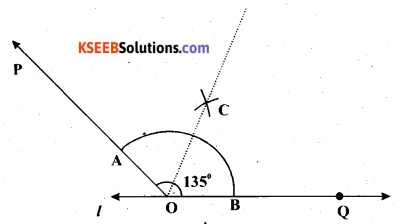The below given steps will be followed to construct an angle of 135° and its bisector.
(1) ∠POQ of 135° measure can be formed on a line l by using the protractor.
(2) Draw an arc of a convenient radius, while taking point O as centre. Let it intersect both rays of angle 135° at point A and B.
(3) Taking A and B as centres, draw arcs of radius more than $$\frac{1}{2}$$ AB in the interior of angle of 135°. Let those intersect each other at C. Join OC.
OC is the required bisector of 135° angle.Question 8.
Draw an angle of 70°. Make a copy of it using only a straight edge and compasses.
Solution:
The below given steps will be followed to construct an angle of 70° measure and its copy.
(1) Draw a line l and mark a point O on it. Place the centre of the protractor at point O and the zero edge along line l.
(2) Mark a point A at 70°. Join OA. OA is the ray making 70° with line l. Draw an arc of convenient radius in the interior of 70° angle, while taking point O as centre. Let it intersect both rays of angle 70° at point B and C.
(3) Draw the line m and mark a point P on it. With the same radius as used before, again draw an arc while taking point P as centre. Let it cut the line m at point D.
(4) Now, adjust the compasses up to the length of BC. With this radius, draw an arc when taking D as centre, which will intersect the previously drawn arc at point E.
(5) Join PE. PE is the required ray which makes the same angle (i.e. 70°) with line m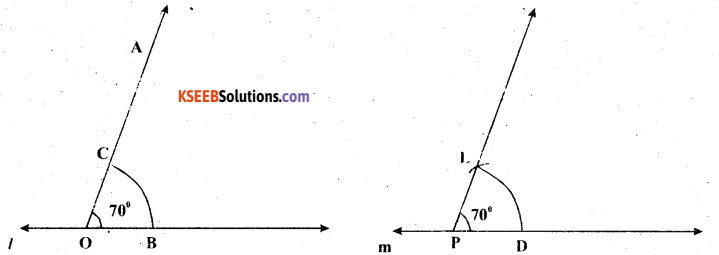Question 9.
Draw an angle of 40°. Copy its supplementary angle.
Solution:
The below given steps will be followed to construct an angle of 40° measure and the copy of its supplementary angle.
(1) Draw a line segment $$\overline{\mathrm{PQ}}$$ and mark a point O on it. Place the centre of the protractor at point O and the zero edge along line segment $$\overline{\mathrm{PQ}}$$.
(2) Mark a point A at 40°. Join OA. OA is the required ray making 40° with $$\overline{\mathrm{PQ}}$$ . ∠POA is the supplementary angle of 40°.
(3) Draw an arc of convenient radius in the interior of ∠POA, while taking point O as centre. Let it intersect both rays of ∠POA at point B and C.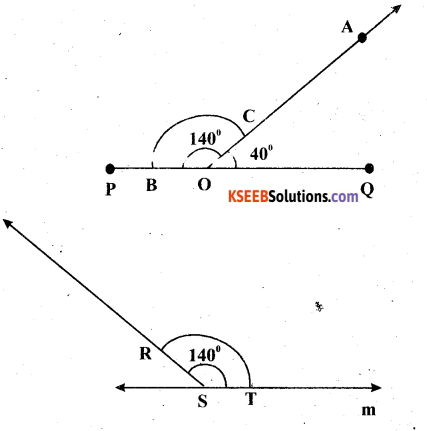(4) Draw a line m and mark a point S on it. With the same radius as used before, again draw an arc while taking point S as centre. Let it cut the line m at point T.
(5) Now, adjust the compasses up to the length of BC. With this radius, draw an arc while taking T as centre, which will intersect the previously drawn arc at point R.
(6) Join RS. RS is the required ray which makes the same angle with line m, as the supplementary of 40° is 140°.

a